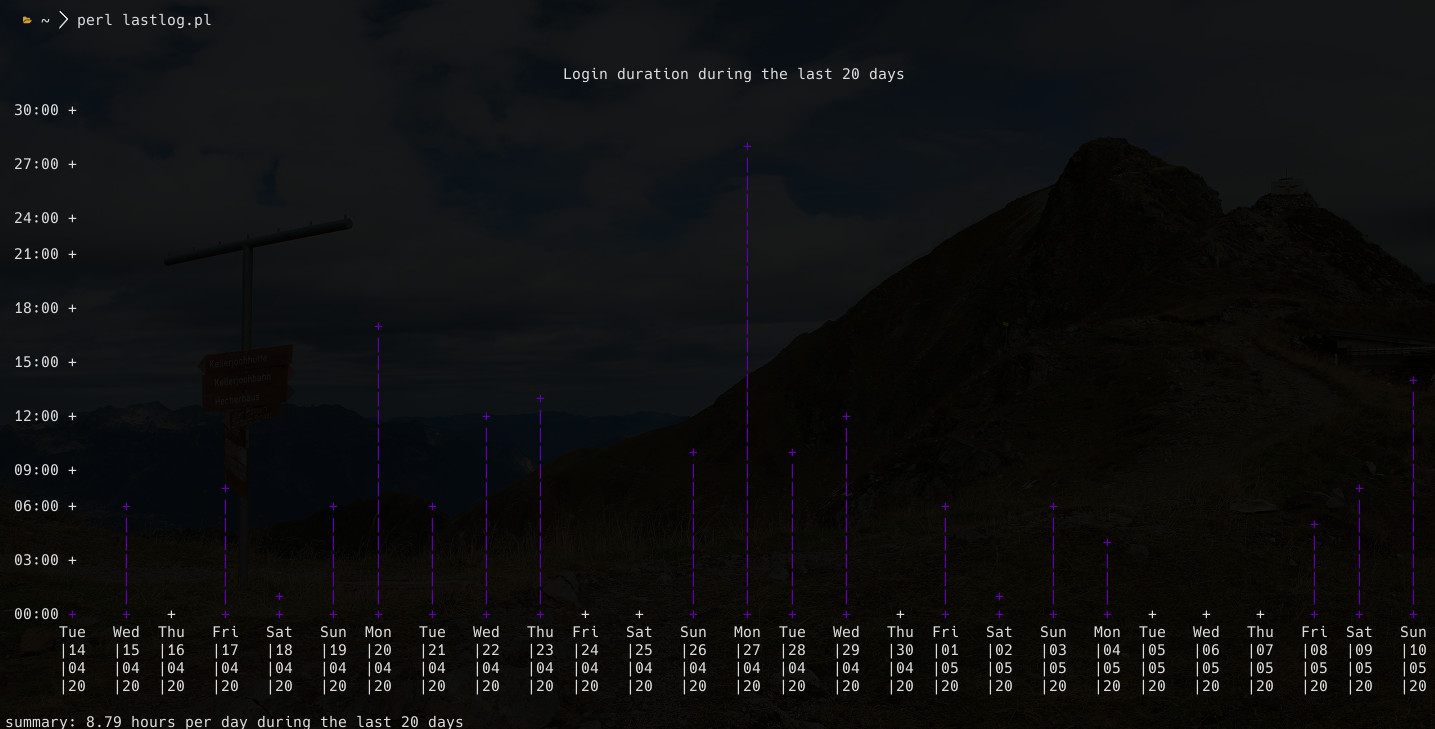gnuplot
configurationother_softwaregnuplot

This is such a mighty tool and it is so sad, that I have not used it more often.

## Uptime graph

gnuplot and perl is 💖``````#!/bin/env perl

my \$days = 20;

# set terminal dumb size 160, 30;
# set grid;
# set format x "%d.%m.%Y";
\$C = <<GNUPLOT;
gnuplot -p -e '
unset border;
unset key;

set colorsequence classic;
set terminal dumb ansirgb noenhanced size 160,37;

set title "Login duration during the last \$days days" font "sans-serif,12";

set xdata time;
set ydata time;
set xtics nomirror rotate 86400 font "sans-serif,8" scale 0;
set ytics nomirror        time  font "sans-serif,8" scale 0;

set autoscale xfix;

set format x "%a\n|%d\n|%m\n|%y";
set format y "%M:%S";

plot
"-" using (timecolumn(1, "%Y-%m-%d")):(timecolumn(2, "%M:%S")) with impulses lc rgb "#6000ad"  lt 6 lw 2,
'
GNUPLOT

my \$total = 0; my \$count = 0;
# TODO: maybe utmpdump /var/log/wtmp is better?
\$O = qx[last --time-format iso reboot -R];
\$O =~ s/(\d{4}-\d{2}-\d{2}).*\d{4}-\d{2}-\d{2}.*\((\d{2}):(\d{2})\)/\$dates{\$1}+=(60.0*\$2)+\$3/eg;
open (W, '|'.\$C) or print \$!;
@list = reverse sort keys(%dates);
foreach (splice @list, 0, \$days) {
my \$d = \$dates{\$_};
my \$m = \$d % 60;
my \$h = (\$d - \$m) / 60;

\$total += \$d;
\$count ++;
# printf(  "%s\t%02d:%02d\n", \$_, \$h, \$m);
printf(W "%s\t%02d:%02d\n", \$_, \$h, \$m);
}
close(W);

# \$total += 7.5 * 60 + 7.5 * 60 + 10 * 60;
printf("summary: %.2f hours per day during the last \$count days\n", \$total / \$days / 60.0);
``````